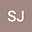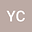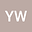Numerical analysis of viscoelastic pipe with variable fractional order model based on shifted Legendre polynomials algorithm
•••• Suhua Jin,
• Yiming Chen,
• Yuanhui Wang
Suhua Jin
Yanshan University

Corresponding Author:1149374915@qq.com

Author ProfileYiming Chen
Yanshan University
Author ProfileYuanhui Wang
Yanshan University
Author Profile## Abstract

In this paper, an valid numerical algorithm is presented to solve variable fractional viscoelastic pipes conveying pulsating fluid in the time domain and analyze dynamically the vortex-induced vibration of the pipes. Firstly, Coimbra variable fractional derivative operators are introduced. Meanwhile, using Hamilton's principle and a nonlinear variable fractional order model, the governing system of equations is established. The unknown functions of the system of equations are approximated with shifted Legendre polynomials. Then, convergence analysis and numerical example investigate the effectiveness and accuracy of the proposed algorithm. Finally, the influences of different parameters on the dynamic response of the viscoelastic pipe are studied. The influencing factors and their ranges of the transient and long-term chaotic states of the pipe are analyzed. In addition, the proposed algorithm shows enormous potentials for solving the dynamics problems of viscoelastic pipes with the variable fractional order models.
19 May 2021Submitted to Mathematical Methods in the Applied Sciences
22 May 2021Assigned to Editor
22 May 2021Submission Checks Completed
01 Jun 2021Reviewer(s) Assigned
09 Nov 2022Editorial Decision: Revise Minor